Author: Oscar Cronquist Article last updated on May 09, 2022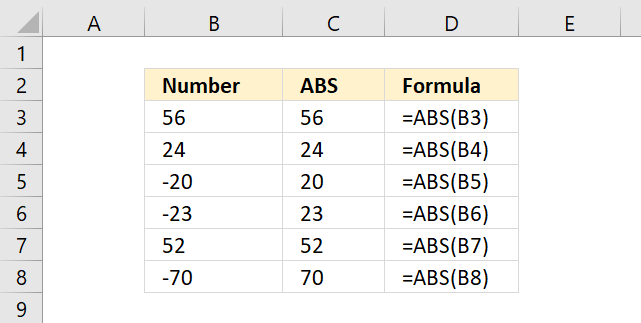Converts negative numbers to positive numbers, in other words, the ABS function removes the sign.

ABS(number)

## 2. ABS Function Arguments

 number The number you want to remove the sign from.

## 3. ABS Function exampleThe ABS function converts a negative number to a positive number, in other words, it removes the sign from a number.

Formula in cell C3:

=ABS(B3)

The ABS function returns #VALUE if you use a text string, however, it returns 1 for boolean value TRUE and 0 (zero) for FALSE.

## 4. How to convert a negative number to positive numbers and vice versa?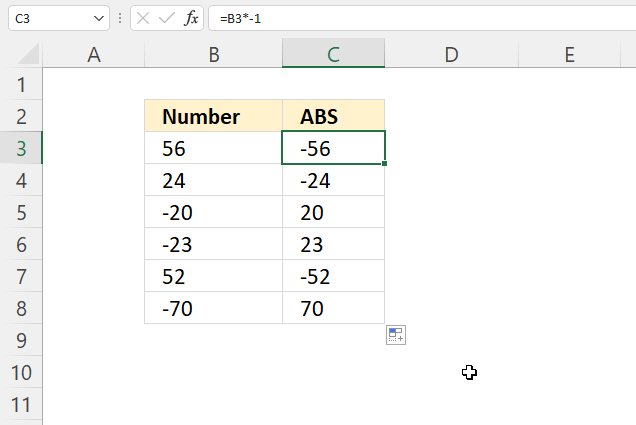The formula in cell C3 converts negative numbers to positive numbers and positive numbers to negative numbers.

Formula in cell C3:

=B3*-1

### 4.1 Explaining formula

B3*-1

returns

56*-1

#### Step 2 - Evaluate formula

56*-1 equals -56.

## 5. How to convert all numbers to negative numbers?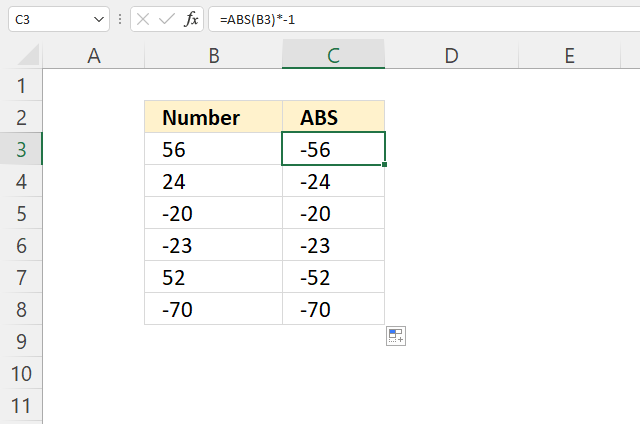Formula in cell C3:

=ABS(B3)*-1

### Explaining formula

ABS(B3)

becomes

ABS(56)

and returns 56.

#### Step 2 - Multiple with -1

The asterisk lets you multiply numbers in an Excel formula.

ABS(B3)*-1

becomes

56*-1

and returns -56.

## 6. Convert negative numbers to positive and calculate sum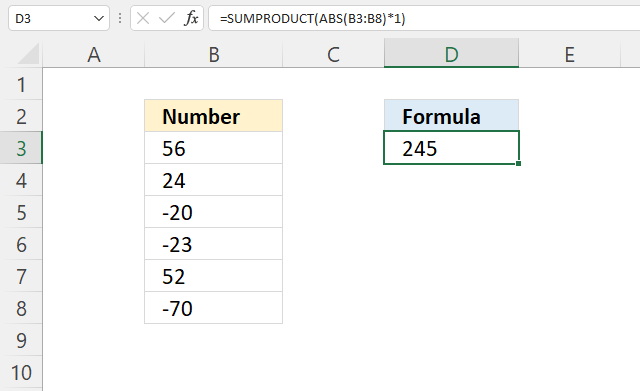The following formula converts negative numbers to positive and then sums the numbers. 56 + 24 + 20 + 23 + 52 + 70 equals 245.

Formula in cell D3:

=SUMPRODUCT(ABS(B3:B8)*1)

### Explaining formula

Excel 365 users can use the following smaller formula:

=SUM(ABS(B3:B8))

#### Step 1 - Convert to absolute numbers

ABS(B3:B8)

becomes

ABS({56; 24; -20; -23; 52; -70})

and returns

{56; 24; 20; 23; 52; 70}.

#### Step 2 - Add absolute numbers and return a total

The SUM function adds numbers and then returns a total.

SUM(ABS(B3:B8))

becomes

SUM({56; 24; 20; 23; 52; 70})

and returns 245.

## 7. Convert negative numbers to positive and calculate average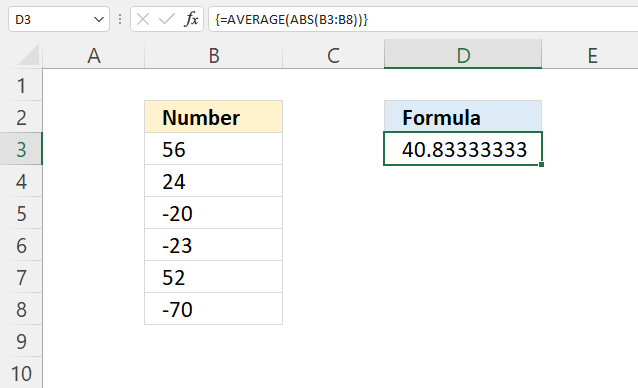The formula in cell D3 removes the negative signs from the numbers in cell range B3:B8 and then calculates an average.

Array formula in cell D3:

=AVERAGE(ABS(B3:B8))

### 7.1 How to enter an array formula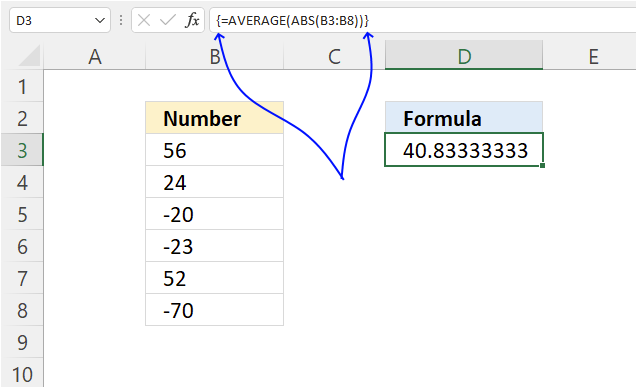1. Copy the array formula above.
2. Double press with the left mouse button on cell D3, a prompt appears.
3. Paste it to cell C3, shortcut keys are CTRL + v.
4. Press and hold CTRL + SHIFT keys simultaneously.
5. Press Enter once.
6. Release all keys.

The formula bar shows a beginning and ending curly bracket, don't enter these characters yourself.

### 7.2 Explaining formula

#### Step 1 - Convert numbers to absolute numbers

ABS(B3:B8)

becomes

ABS({56; 24; -20; -23; 52; -70})

and returns

{56; 24; 20; 23; 52; 70}.

#### Step 2 - Calculate an average

The AVERAGE function calculates the average of numbers in a cell range.

AVERAGE(number1[number2], ...)

AVERAGE (ABS(B3:B8))

becomes

AVERAGE ({56; 24; 20; 23; 52; 70})

and returns approx. 40.83

## 8. Convert negative numbers to positive and calculate median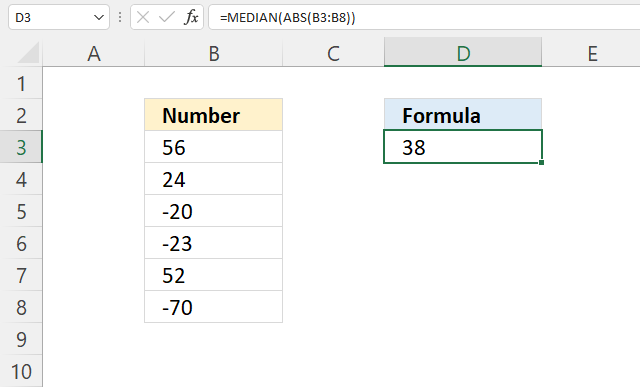Array formula in cell D3:

=MEDIAN(ABS(B3:B8)*1)

How to enter an array formula

### Explaining array formula

#### Step 1 - Convert to absolute numbers

ABS(B3:B8)

becomes

ABS({56; 24; -20; -23; 52; -70})

and returns

{56; 24; 20; 23; 52; 70}.

#### Step 2 - Calculate median

The MEDIAN function calculates the median based on a group of numbers. The median is the middle number of a group of numbers.

If the group contains an even number of numbers the MEDIAN function calculates the average of the two numbers in the middle.

MEDIAN(ABS(B3:B8))

becomes

MEDIAN({56; 24; 20; 23; 52; 70})

and returns 38. 52 + 24 equals 76. 76 / 2 equals 38.